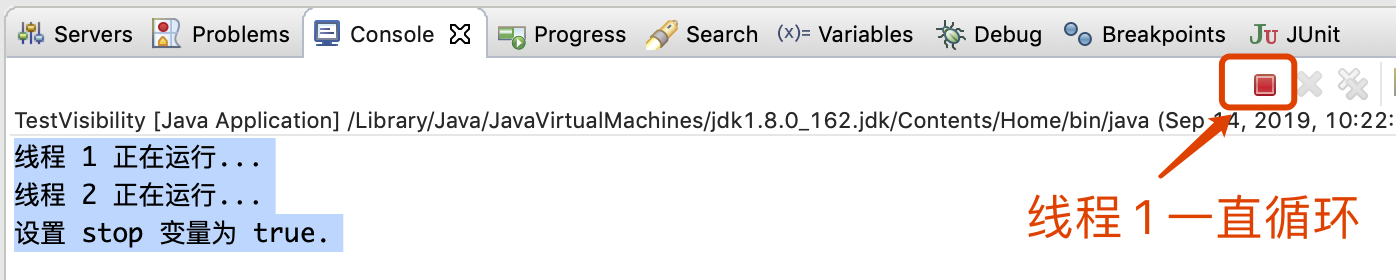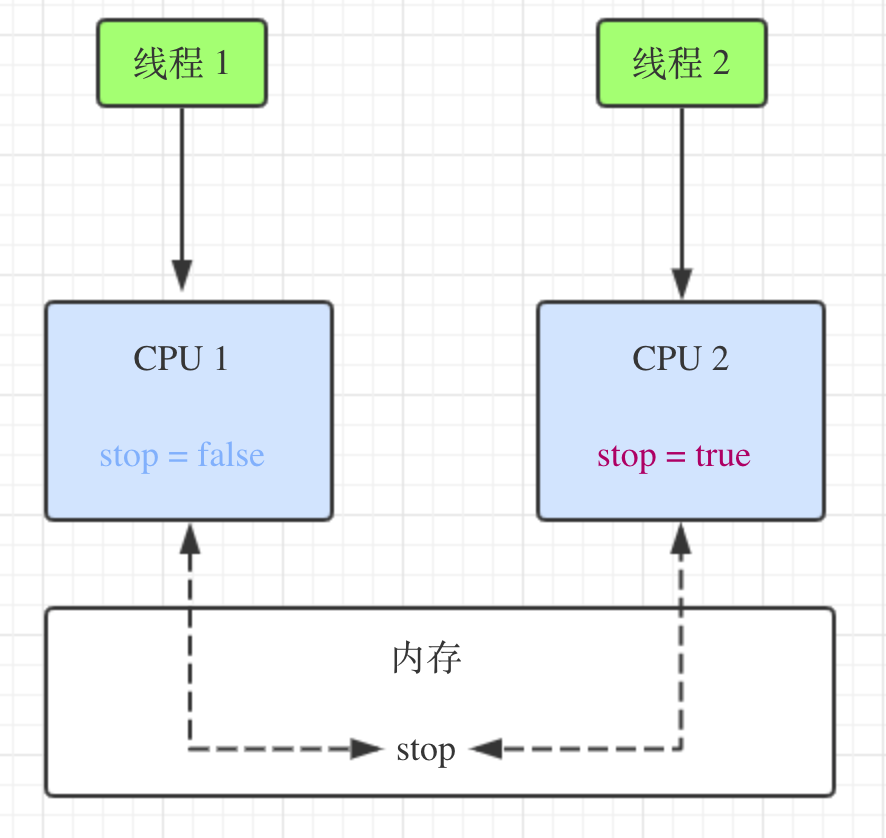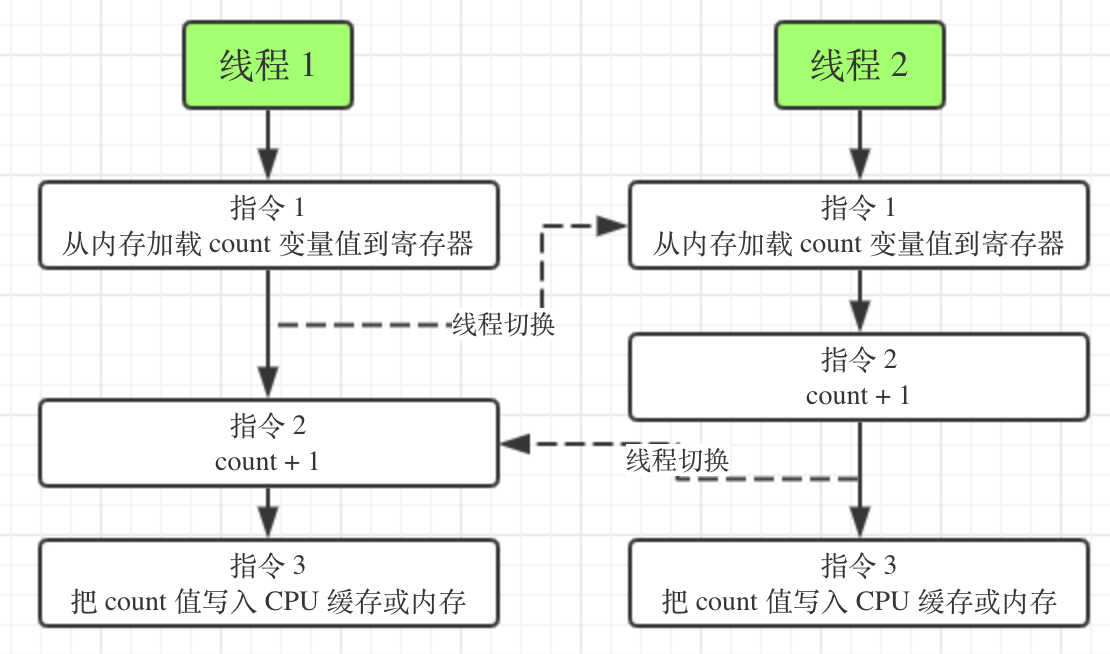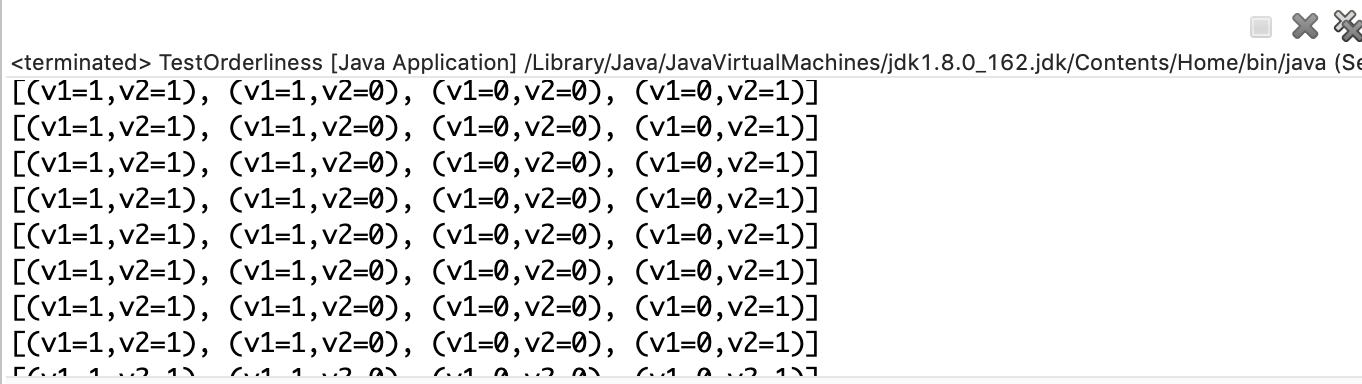## 导致并发程序出问题的根本原因是什么？

2019-09-14

CPU、内存、IO 设备的读写速度差异巨大，表现为 CPU 的速度 > 内存的速度 > IO 设备的速度。

• 计算机体系结构，给 CPU 增加了缓存，均衡 CPU 和内存的速度差异
• 操作系统，增加了进程与线程，分时复用 CPU，均衡 CPU 和 IO 设备的速度差异
• 编译器，增加了指令执行重排序，更好地利用缓存，提高程序的执行速度

1、 CPU 缓存，在多核 CPU 的情况下，带来了可见性问题

``````package constxiong.concurrency.a014;

/**
* 测试可见性问题
* @author ConstXiong
*/
public class TestVisibility {

//是否停止 变量
private static boolean stop = false;

public static void main(String[] args) throws InterruptedException {
//启动线程 1，当 stop 为 true，结束循环
new Thread(() -> {
System.out.println("线程 1 正在运行...");
while (!stop) ;
System.out.println("线程 1 终止");
}).start();

//休眠 10 毫秒
Thread.sleep(10);

//启动线程 2， 设置 stop = true
new Thread(() -> {
System.out.println("线程 2 正在运行...");
stop = true;
System.out.println("设置 stop 变量为 true.");
}).start();
}

}
``````2、操作系统对当前执行线程的切换，带来了原子性问题

``````package constxiong.concurrency.a014;

/**
* 测试原子性问题
* @author ConstXiong
*/
public class TestAtomic {

//计数变量
static volatile int count = 0;

public static void main(String[] args) throws InterruptedException {
//线程 1 给 count 加 10000
Thread t1 = new Thread(() -> {
for (int j = 0; j < 10000; j++) {
count++;
}
System.out.println("thread t1 count 加 10000 结束");
});

//线程 2 给 count 加 10000
Thread t2 = new Thread(() -> {
for (int j = 0; j < 10000; j++) {
count++;
}
System.out.println("thread t2 count 加 10000 结束");
});

//启动线程 1
t1.start();
//启动线程 2
t2.start();

//等待线程 1 执行完成
t1.join();
//等待线程 2 执行完成
t2.join();

//打印 count 变量
System.out.println(count);
}

}
``````

``````thread t2 count 加 10000 结束
thread t1 count 加 10000 结束
11377``````

Java 代码中 的 count++ ，至少需要三条 CPU 指令：

• 指令 1：把变量 count 从内存加载到 CPU 的寄存器
• 指令 2：在寄存器中执行 count + 1 操作

• 指令 3：+1 后的结果写入 CPU 缓存 或 内存3、编译器指令重排优化，带来了有序性问题

``````package constxiong.concurrency.a014;

import java.util.HashMap;
import java.util.HashSet;
import java.util.Map;
import java.util.Set;

/**
* 测试有序性问题
* @author ConstXiong
*/
public class TestOrderliness {

static int x;//静态变量 x
static int y;//静态变量 y

public static void main(String[] args) throws InterruptedException {
Set<String> valueSet = new HashSet<String>();//记录出现的结果的情况
Map<String, Integer> valueMap = new HashMap<String, Integer>();//存储结果的键值对

//循环 1000 万次，记录可能出现的 v1 和 v2 的情况
for (int i = 0; i < 10000000; i++) {
//给 x y 赋值为 0
x = 0;
y = 0;
valueMap.clear();//清除之前记录的键值对
Thread t1 = new Thread(() -> {
int v1 = y;//将 y 赋值给 v1 ----> Step1
x = 1;//设置 x 为 1  ----> Step2
valueMap.put("v1", v1);//v1 值存入 valueMap 中  ----> Step3
}) ;

Thread t2 = new Thread(() -> {
int v2 = x;//将 x 赋值给 v2  ----> Step4
y = 1;//设置 y 为 1  ----> Step5
valueMap.put("v2", v2);//v2 值存入 valueMap 中  ----> Step6
});

//启动线程 t1 t2
t1.start();
t2.start();
//等待线程 t1 t2 执行完成
t1.join();
t2.join();

//利用 Set 记录并打印 v1 和 v2 可能出现的不同结果
valueSet.add("(v1=" + valueMap.get("v1") + ",v2=" + valueMap.get("v2") + ")");
System.out.println(valueSet);
}
}

}
``````v1=0,v2=0 的执行顺序是 Step1 和 Step 4 先执行

v1=1,v2=0 的执行顺序是 Step5 先于 Step1 执行

v1=0,v2=1 的执行顺序是 Step2 先于 Step4 执行

v1=1,v2=1 出现的概率极低，就是因为 CPU 指令重排序造成的。Step2 被优化到 Step1 前，Step5 被优化到 Step4 前，至少需要成立一个。

ConstXiong 备案号：苏ICP备16009629号-3